Date: Fri, 15 Jan 1999 11:48:51 EST
To: QandQ@MathCentral.uregina.ca

Hello my name is Kirk from Scarborough, Ontario.

I have been out of a formal education system for thirty years. I program microcontrollers in my spare time. I have built a temperature sensing device ready to go but, thermistors are very non-linear. I do know that there is a way to calculate the input condition of the thermistor and display the correct temperature in degrees C. I am sending a file to show my progression so far.

(T is temperature in degrees C and R is resistance in Kohms.)

T R T R T R T R -50 325.2 -10 42.45 30 8.313 70 2.229 -45 247.5 -5 33.89 35 6.941 75 1.924 -40 188.4 0 27.28 40 5.828 80 1.669 -35 144.0 5 22.05 45 4.912 85 1.451 -30 111.3 10 17.96 50 4.161 90 1.366 -25 86.39 15 14.68 55 3.537 95 1.108 -20 67.74 20 12.09 60 3.021 100 .9735 -15 53.39 25 10.00 65 2.589 105 .8575 110 .7579
Kirk

Hi Kirk

I plotted the temperature (T) and resistance (R) data that you sent and indeed the relationship is non-linear as you indicated.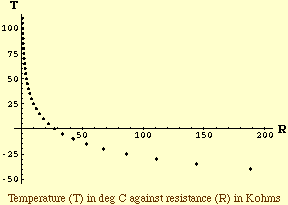One way to approach this problem is to attempt to linearize it and then use linear regression to fit a line. To do so you need to recognize the functional form from the shape of the plotted data. The plot of your data resembles a log curve so my initial conjecture is that the functional form is T = A + B Log(R) where A and B are constants and Log is the natural log function. (You could just as easily use the common log, that is the log base 10.) I then took the log of each R value and plotted Log(R) against T.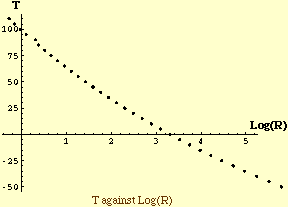This plot does appear approximately linear and performing a linear fit (linear regressiion) produces T = g(R)  = 91.97 - 26.43 Log(R). Plotting this function with the data points shows a reasonable fit but I think we can do better.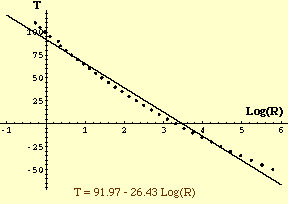For each data point measure the vertical distance between the point and the line, that is for each data point compute T-g(R). Now plot T-g(R) against Log(R) to get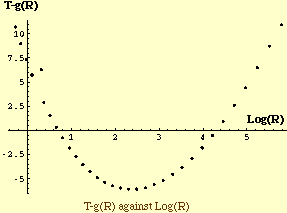This plot resembles a parabola T - g(R) = C + D Log(R) + E Log(R)^2 and hence, collecting like terms, perhaps a better functional form is T = P + Q Log(R) + R Log(R)^2. This time a two variable linear fit produces T = 98.65 - 36.20 Log(R) + 1.86 Log(R)^2 and its graph indicates a much better fit to the data.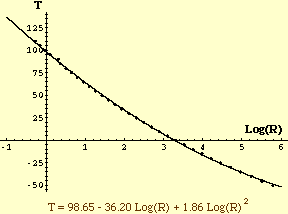I hope this helps
Harley

Go to Math Central